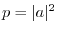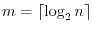Subsections

# Miscellaneous

## quantum_gate_counter

 extern int quantum_gate_counter(int inc);

libquantum keeps track of the number of all quantum operations run during the execution of a program. This functions allows you to increase the counter by inc steps. The counter can be set to zero by passing a negative increment. The function always returns the current value of the counter.

## quantum_prob

 extern float quantum_prob(COMPLEX_FLOAT a);

The probability for a basis state can be determined with this function. The argument is a complex number, usually a probability amplitude of a basis state. The return value is the square of the absolute value of the complex number, i.e..

Of course, this function is not available on a real quantum computer, since the quantum state is not observable.

## quantum_getwidth

 extern int quantum_getwidth(int n);

To determine the number of qubits required to encode a classical number, this function can be used. The return value is.

## quantum_get_version

 extern const char * quantum_get_version();

Returns a string containing the version of libquantum.

## quantum_print_timeop

 extern void quantum_print_timeop(int width, void f(quantum_reg *));

Prints the time evolution operator acting on width qubits by running the operations within the function f. The function is called with a single argument, which is a pointer to a quantum register.